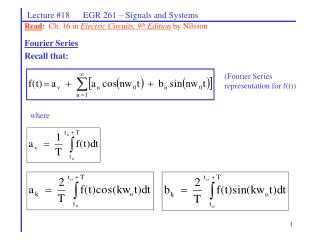Download PresentationRead : Ch. 16 in Electric Circuits, 9 th Edition by NilssonRead : Ch. 16 in Electric Circuits, 9 th Edition by Nilsson - PowerPoint PPT Presentation

Download PresentationRead : Ch. 16 in Electric Circuits, 9 th Edition by Nilsson
An Image/Link below is provided (as is) to download presentation

Download Policy: Content on the Website is provided to you AS IS for your information and personal use and may not be sold / licensed / shared on other websites without getting consent from its author. While downloading, if for some reason you are not able to download a presentation, the publisher may have deleted the file from their server.

- - - - - - - - - - - - - - - - - - - - - - - - - - - E N D - - - - - - - - - - - - - - - - - - - - - - - - - - -
Presentation Transcript

1. Lecture #18 EGR 261 – Signals and Systems Read: Ch. 16 in Electric Circuits, 9th Edition by Nilsson Fourier Series Recall that: (Fourier Series representation for f(t)) where

2. v(t) T Vm t - 1 0 1 2 3 Lecture #18 EGR 261 – Signals and Systems Example: A) Find the Fourier Series for v(t) shown below.

3. v(t) T 0.5Vm t 1 0 1 2 3 -0.5Vm Lecture #18 EGR 261 – Signals and Systems • Write out the first several terms in the Fourier Series. • Discuss how some of the results above could be determined by symmetry. The symmetry in v(t) above is clearer if the average value is removed as shown below. We will soon investigate the effects of symmetry on a Fourier Series. If we had already done so, we could have predicted that ak = 0 for all k and that bk = 0 for k = even. This is because the waveform above has: Half-wave symmetry – thus it has no even harmonics Odd symmetry – thus it has no cosine terms (i.e., ak = 0)

4. Lecture #18 EGR 261 – Signals and Systems • The effect of symmetry in a Fourier Series • 4 types of symmetry will be considered: • Even-function symmetry • Odd-function symmetry • Half-wave symmetry • Quarter-wave symmetry

5. f(t) f(t) t t 0 0 f(t) = Kcos(wt) f(t) t t 0 0 Lecture #18 EGR 261 – Signals and Systems Even-function symmetry A function is even if f(t) = f(-t). This type of symmetry depends on where t = 0. The y-axis is the axis of symmetry (the waveform is mirrored about the y-axis). Examples:

6. Lecture #18 EGR 261 – Signals and Systems Even-function symmetry

7. -T f(t) 2 +T 2 t 0 Lecture #18 EGR 261 – Signals and Systems Even-function symmetry - Proof Show that the results above apply to any general function f(t), such as the one shown below, which has even symmetry. Determine expressions for bk and ak in general to verify the results above. (Integrate from –T/2 to 0 and 0 to T/2 and then use a substitution on the first integral: t = -x so dt = -dx and when t = T/2 then x = -T/2.)

8. f(t) t f(t) = Ksin(wt) t f(t) t Lecture #18 EGR 261 – Signals and Systems Odd-function symmetry A function is odd if f(t) = -f(-t). This type of symmetry depends on where t = 0. It is often helpful to remove the average (DC) value to see the symmetry. This symmetry is present if the waveform is mirrored and flipped about the y-axis. Examples: (If Av0, sketch the waveform with the DC level removed.)

9. Lecture #18 EGR 261 – Signals and Systems Odd-function symmetry

10. f(t) axis of symmetry T T 2 2 0 t Lecture #18 EGR 261 – Signals and Systems Half-wave symmetry A function has half-wave symmetry if f(t) = -f(t – T/2). In other words, if you shift the function one-half period and invert it, it is identical to the original function. This type of symmetry does not depend on where t = 0. This symmetry is present if one half of a period is a mirrored image of the other half. Example: Note that the waveform below is neither even nor odd, but has half-wave symmetry. If the waveform were shifted, it could also have either even or odd symmetry.

11. Lecture #18 EGR 261 – Signals and Systems Half-wave symmetry So, a periodic function with half-wave symmetry has zero average and contains only odd harmonics.

12. v(t) axis of half symmetry Vm Vm 2 t 0 T T 3T T 2 4 4 axes of quarter symmetry Lecture #18 EGR 261 – Signals and Systems Quarter-wave symmetry To have quarter-wave symmetry, there must also be half-wave symmetry. If a waveform with half-wave symmetry also is symmetric about the center of each half-cycle, then it also has quarter-wave symmetry. Example: The waveform below has both half-wave and quarter-wave symmetry. Does it have any other type of symmetry?

13. Lecture #18 EGR 261 – Signals and Systems Quarter-wave symmetry

14. v(t) Vm Vm 2 t 0 T T 3T T 2 4 4 Lecture #18 EGR 261 – Signals and Systems Example: Find the Fourier Series representation for v(t) below if Vm = 10V and T = 2ms.

15. v(t) 10V 5V t 0 2 4 6 10 12 Lecture #18 EGR 261 – Signals and Systems Example: Find the Fourier Series representation for v(t) below.